# How to calculate the induced charge in an electric field

stanlee
Homework Statement:
The aim of project is to measure the electric field with field mill. These two semi-cylindrical conductor rotating in electric field, how can I calculate the induced charge and the current.
Relevant Equations:
Below the picture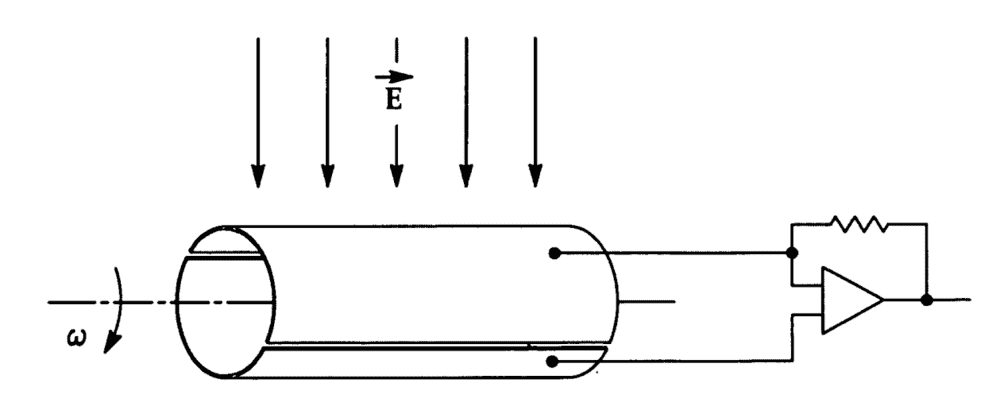Maybe I should use this?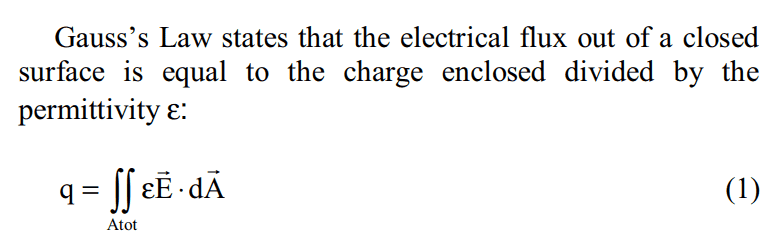stanlee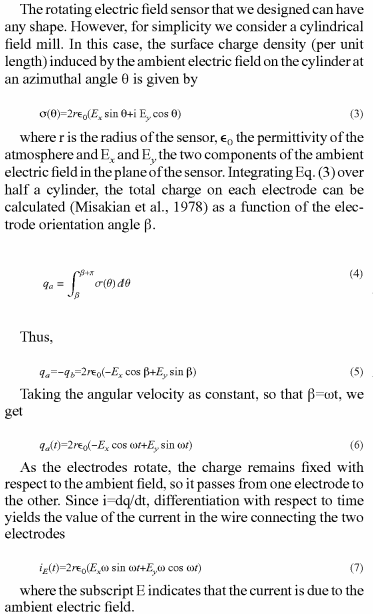I don't know whether this is correct.

Homework Helper
Gold Member
You essentially have the problem of finding the induced surface charge density on a long, cylindrical conductor placed in a uniform E-field oriented perpendicular to the axis of the cylinder. This is a fairly standard boundary value problem in electrostatics using separation-of-variables in Laplace's equation. The solution will give you something similar to what is given in equation (3):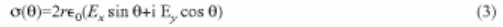However, the presence of ##i## in this equation looks odd to me. But, thankfully, this ##i## disappears in equation (5).

##\sigma(\theta)## is supposed to be the "charge per unit length". I'm not sure how to interpret this. If you include an additional factor of the length, ##L##, of the cylinder, then the equation (without the ##i##) would be

##\sigma(\theta) = 2rL\epsilon_0\left(E_x \sin\theta + E_y \cos \theta \right)##

I believe this now represenst the charge per unit angle ##\theta##. Then it would make sense to write ##q_a = \int \sigma(\theta) d \theta## as in equation (4).

stanlee
You essentially have the problem of finding the induced surface charge density on a long, cylindrical conductor placed in a uniform E-field oriented perpendicular to the axis of the cylinder. This is a fairly standard boundary value problem in electrostatics using separation-of-variables in Laplace's equation. The solution will give you something similar to what is given in equation (3):

However, the presence of ##i## in this equation looks odd to me. But, thankfully, this ##i## disappears in equation (5).

##\sigma(\theta)## is supposed to be the "charge per unit length". I'm not sure how to interpret this. If you include an additional factor of the length, ##L##, of the cylinder, then the equation (without the ##i##) would be

##\sigma(\theta) = 2rL\epsilon_0\left(E_x \sin\theta + E_y \cos \theta \right)##

I believe this now represenst the charge per unit angle ##\theta##. Then it would make sense to write ##q_a = \int \sigma(\theta) d \theta## as in equation (4).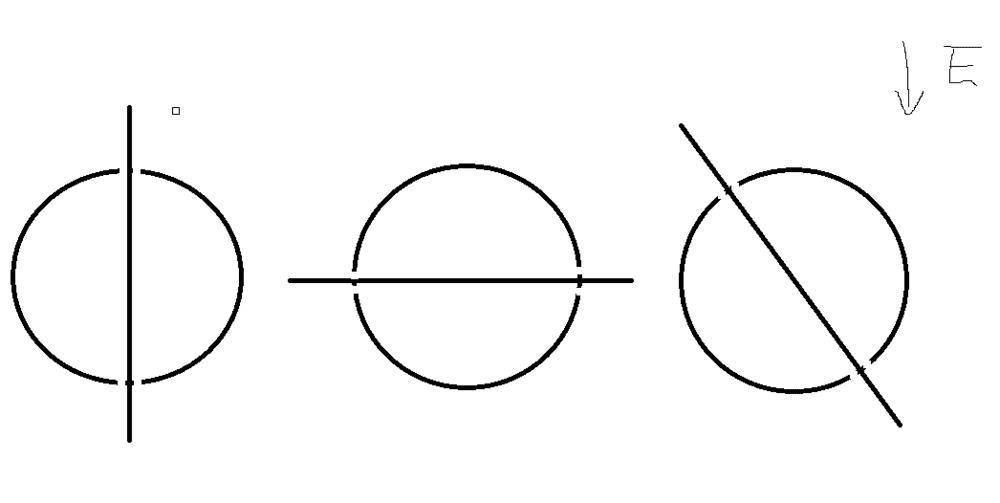If there is only a vertical electric field, can you show me the charge distributed in there surface? And is the current equals to zero in first image? Why?

Homework Helper
Gold Member
The two half-cylinders are connected together by the external circuit such that you can consider the entire cylinder to be a single conductor at one potential. (This assumption seems to lead to the expressions given in the document that you posted.) So, the charge distribution at any instant is just the charge distribution of a full cylinder in the electric field. So, in your first image, ignore for the moment the split in the cylinder. Qualitatively, how would the charge be distributed on the surface? Once you see this, then you should be able to see whether each half-cylinder has any net charge in the first image. Repeat for the other two images. Then we can think about whether or not there is any current for the three images.

stanlee
The two half-cylinders are connected together by the external circuit such that you can consider the entire cylinder to be a single conductor at one potential. (This assumption seems to lead to the expressions given in the document that you posted.) So, the charge distribution at any instant is just the charge distribution of a full cylinder in the electric field. So, in your first image, ignore for the moment the split in the cylinder. Qualitatively, how would the charge be distributed on the surface? Once you see this, then you should be able to see whether each half-cylinder has any net charge in the first image. Repeat for the other two images. Then we can think about whether or not there is any current for the three images.
Then every images should have negtive charge in the up semi-cylindrical and positive in the bottom semi-cylindrical.

Homework Helper
Gold Member
Yes.

stanlee
Yes.Can you derive this formula in detail or some website I can learn by myself? Thank you very much!

Homework Helper
Gold Member
Have you studied solving Laplace's equation in various coordinate systems using separation of variables? If so, look at the first page here . The general solution in polar coordinates is at the bottom of the page. You need to apply boundary conditions to determine the various constants ##A_n, B_n, C_n,## and ##D_n##.